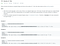# Javascript Bitwise Operators and Algo problem

As a continuation of my study of algorithms, I recently ran into this challenge on LeetCode. When I first read the question below, I was puzzled. I didn’t even know where to begin. Luckily for me, Google exists. I soon read more about binary code and bitwise operators.LeetCode Problem 191

I won’t dive too deep into how binary code works since it is a pretty well documented and can easily be found online. I will however, discuss some of the operators and how I was able to use them in my solution to this practice problem.

1. & or “AND” operator returns the matching result of two inputs. For example, if we have 5 &1 (0101 & 0001) our output or result would be the far right matching 1. So the result from using this operator is 1in decimal or 0001 in binary.
2. | or “OR” operator sets each bit to 1 if one of the two bits is 1. If we have 5 | 1 or (0101 | 0001) we would expect and output of 5 or 0101.
3. ^ or “XOR” sets the outlying bit to 1. For example, 5^ 1 (0101 ^ 0001) would return the non matching 1 bit ==> 0100 = 4.
4. ~ or “NOT” inverts the bits. So, 0001 would yield 1110.
5. << or “Zero Fill Left Shift” shifts all bits to the left by adding zeros in from the right. In this case of 5<<1 or (0101<< 1) would return 1010 or 10.
6. >> or “Signed right Shift” shifts right by pushing copies of the most left bit from the left and removing the rightmost bit. In this example, 5>>1 or (0101>>1) we get 0010 or 2.
7. >>> or “Zero Fill right” shifts right by pushing zeros in from the left. For example, 5>>>1 or ( 01010>>>1) would return 0010 or 2.

Great! Now that we have learned a little more about how the bitwise operators work we are ready to dive into our solution to this problem!

# Solution:

I decided to begin with a sum initialized to 0. From here, we set a while loop with the conditional of the input not equal to 0. Next, we use the addition assignment operator to continue the sum of the bits while our current input is used against 1 with the & operator. Next, while we hold the new value of the input we can now Zero Fill Right Shift (>>>) allowing for the most right bit to fall off. We do this until all the 1 bits are counted and we are returned an integer representing the number of 1 bits in the 32 bit string!

Resources:

Full-Time Software Engineering Student at Flatiron School, avid golfer, and wannabe chef.

## More from Seyi Kanagui

Full-Time Software Engineering Student at Flatiron School, avid golfer, and wannabe chef.